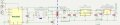# Push pull transformer

#### Unknown1991

Joined Oct 17, 2022
2
Hi,
Please, can you help me to solve these questions. I didn’t work with push pull converter before so I am trying to understand the concept of how it works but still I couldn’t figure out how to answer the below questions. (The figure of the circuit is attached)
What is the maximum current that we can supply with this design?
What is the maximal and minimal turns ratio of the transformer to make sure that the power is reliable?#### BobTPH

Joined Jun 5, 2013
6,035
Is this homework? Ut sure sounds like it.

#### Irving

Joined Jan 30, 2016
3,180
What is the maximum current that we can supply with this design?

I suspect the transformer is irrelevant as the 5v rail from that 1st regulator is limited to 150mA and the 3.3v to 200mA (though in reality its input current limited by whatever is left from the 5v rail).

The Wurth reference design for that transformer & TI chip combo gives 5v @ 350mA but TI's worked example in their datasheet shows 5v @ 100mA because that was the example's requirement.
The Maxim MAX13253 reference design uses HALO transformers but will give a similar output for a 1:1.7 ratio transformer.

What is the maximal and minimal turns ratio of the transformer to make sure that the power is reliable?

What do you mean by 'reliable'? Turns ratio will only impact input voltage range. These transformers & chips assume a 3.3v DC input as they are intended to provide isolated supplies for common-mode protection, and are not recommended for operation off batteries for example. They should be OK for 3.3 +/- 5% at 200mA output but you'll have to run the math to be 100% sure.

#### Unknown1991

Joined Oct 17, 2022
2
Thank you all.
This how I calculated the maximal and minimal turns ratio of the transformer:

SN6505A is not used since it has DNP (do not place) as a comment.

TC2186 is used to output 5v.
According to the datasheet of TC2186 Vin max = 6v and Vin min should equal 5v plus the drop out voltage 210mv (TC2186 datasheet), then Vin min = 5.21v.
The Vin min and Vin max of TC2186 should be supplied by MAX13253, so Vout max =6v andVout min =5.21v.
According to the MAX13253 Vout= ; Vin = 3.3vaccording to the example; VD = 0.300v (worst case) according to SBR80520LT1G datasheet.
Maximum turn ratio n min = (6-0.3)/3.3 = 1.7.
Minimum turn ratio n min = (5.21-0.3)/3.3 = 1.49.